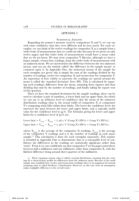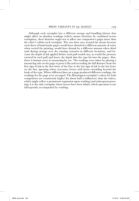# University of Virginia Library

Search this document

128#### Statistical Analysis

Regarding the printer's measure used by compositors X and Y, we can say with some confidence that they were different and by how much. For each exemplar, we can think of the twelve readings for compositor X as a sample from a wider body of measurements that we could not take (because he set prose on only twelve pages) and this wider body of measurements would have a mean value that we do not know. We may treat compositor Y likewise, although we have a larger sample, twenty-four readings, from the wider body of measurements with an unknown mean. We are interested in the difference between the two unknown means, and can use the statistic called 'the difference in the sample means' to comment upon it. In Appendix One, the numerical means of the sample for each exemplar are given: this is simply the sum of the readings divided by the number of readings, twelve for compositor X and twenty-four for compositor Y. An expression of how widely or narrowly the readings are spread around the mean is called the 'standard deviation' (here SD). This is calculated by squaring each reading's difference from the mean, summing these squares and then dividing that sum by the number of readings, and finally taking the square root of this quotient.

Once we have the standard deviations for the sample readings, these can be used to calculate a pair of numbers, a lower limit and an upper limit, for which we can say to an arbitrary level of confidence that the mean of the unknown distribution readings (that is, the actual width of compositor X or compositor Y's composing stick) falls within those limits. The lower the confidence level, the narrower the span between the lower and upper limits, and a typically useful value for the confidence level is 95%. The formulas giving the lower and upper limits for a confidence level of 95% are:
Lower limit = Ymean – Xmean – (1.96 × √ (Comp X's SD2/n + Comp Y's SD2/n))
Lower limit = Ymean – Xmean + (1.96 × √ (Comp X's SD2/n + Comp Y's SD2/n))
where Xmean is the average of the compositor X readings, Ymean is the average of the compositor Y readings and n is the number of readings in each man's sample.11 This calculation is done for each exemplar separately. This statistic is included in Appendix One to demonstrate that to a reasonable level of confidence the differences in the readings are statistically significant rather than 'noise'. From it we can confidently say that compositor Y set his pages somewhere between half a millimetre and one millimetre wider than compositor X, with the likeliest difference being around three-quarters of the millimetre.

129Although each exemplar has a different storage and handling history that might affect its absolute readings (which cannot therefore be combined across exemplars), these histories ought not to affect one compositor's pages more than the other's within each exemplar. The raw data vary around the means because each sheet of hand-made paper would have absorbed a different amount of water when wetted for printing, would have shrunk by a different amount when dried (and during storage over the ensuing centuries in different locations), and because the depth of ink applied before each pull would vary, as would the pressure exerted by each pull and hence the depth that the type bit into the paper. Also, there is human error in measuring by eye. The readings were taken by placing a measuring rule on the page to press it flat and recording the full distance from the first sign of ink in the first letter of the line to the last sign of ink in the last letter on the line, ignoring where necessary letters with kerns extending beyond the body of the type. Where different lines on a page produced different readings, the readings for the page were averaged. The Huntington exemplar's values for both compositors are consistently higher (by about half a millimetre) than the others, which might reflect a permanent expansion upon washing (and subsequent pressing); it is the only exemplar whose leaves have been inlaid, which operation is not infrequently accompanied by washing.

130[11.]

These equations assume what is called a normal distribution, meaning that the readings fit a characteristic profile for differences from the unknown mean. Because of the small sample sizes available here (twelve and twenty-four readings) there is an argument for instead assuming what is called Student's t-distribution. However, in this case such an assumption makes negligible difference to the overall result.